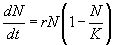index: click on a letter A B C D E F G H I J K L M N O P Q R S T U V W X Y Z A to Z index index: subject areas numbers & symbols sets, logic, proofs geometry algebra trigonometry advanced algebra & pre-calculus calculus advanced topics probability & statistics real world applications multimedia entrieswww.mathwords.com about mathwords website feedback

 Logistic Growth A model for a quantity that increases quickly at first and then more slowly as the quantity approaches an upper limit. This model is used for such phenomena as the increasing use of a new technology, spread of a disease, or saturation of a market (sales). The equation for the logistic model is. Here, t is time, N stands for the amount at time t, N0 is the initial amount (at time 0), K is the maximum amount that can be sustained, and r is the rate of growth when N is very small compared to K. Note: The logistic growth model can be obtained by solving the differential equationSee also

 this page updated 19-jul-17 Mathwords: Terms and Formulas from Algebra I to Calculus written, illustrated, and webmastered by Bruce Simmons Copyright © 2000 by Bruce Simmons All rights reserved